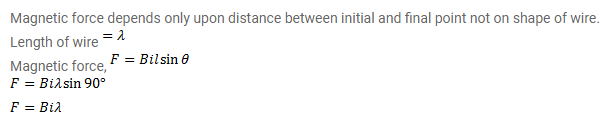# A wire, carrying a current i,

Question:

A wire, carrying a current $i$, is kept in the X-Y plane along the curve $y=A \sin \left(\frac{2 \pi}{\lambda} x\right)$ A magnetic field B exists in the $z-$

direction. Find the magnitude of the magnetic force on the portion of the wire between $x=0$ and $x=\lambda$.

Solution: